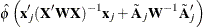### Confidence Intervals for Smoothers

Buja, Hastie, and Tibshirani (1989) showed that each smoothing function estimate from the backfitting algorithm is the result of a linear mapping applied to the working response, if the backfitting algorithm converges.

The smoothing function estimate can be expressed as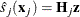where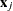is the jth covariate andis the adjusted dependent variable that is formed in the local scoring algorithm. If the errors are independent and identically distributed, then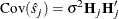where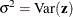.

However, direct computation of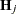is formidable within the backfitting framework. Hastie and Tibshirani (1990) proposed using each individual smoothing matrix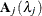as a substitute for the linear operatorwhen computing confidence intervals. In the GAM procedure, curvewise confidence intervals for smoothing splines and pointwise confidence intervals for loess are provided in the output data set.

#### Curvewise Confidence Interval for Smoothing Spline Smoothers

Viewing the spline model as a Bayesian model, Wahba (1983) proposes Bayesian confidence intervals for smoothing spline estimates as: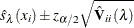where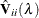is the ith diagonal element of the Bayesian posterior covariance matrix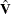and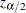is the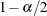quantile of the standard normal distribution. The confidence intervals are interpreted as intervals across the function as opposed to pointwise intervals.

Suppose that you fit a spline estimate to experimental data that consist of a true function f and a random error term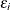. In repeated experiments, it is likely that about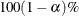of the confidence intervals cover the corresponding true values, although some values are covered every time and other values are not covered by the confidence intervals most of the time. This effect is more pronounced when the true response curve or surface has small regions of particularly rapid change.

In the GAM procedure, let the smoothing matrix for the nonlinear part of the jth spline term be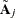after the linear part is separated out from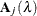. The Bayesian posterior variance for the nonlinear part is computed as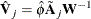where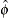is the dispersion parameter estimate and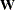is the weight matrix from the final local scoring iteration. If you specify UCLM, LCLM, ADIAG, and STD options in the OUTPUT statement, the statistics are derived based on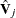.

When you request both the ADDITIVE and CLM suboptions in the PLOTS=COMPONENTS option, each of the smoothing component plots displays a confidence band for the total contribution of each smoothing spline smoother. The confidence band is derived from the total variance that is contributed by both linear and nonlinear parts by the jth term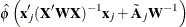#### Pointwise Confidence Interval for Loess Smoothers

As shown in Cleveland, Devlin, and Grosse (1988), the smoothing matrix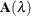for a loess smoother is asymmetric. The confidence intervals are computed as follows:whereis the ith diagonal element of the covariance matrixandis thequantile of the standard normal distribution.

In the GAM procedure, let the smoothing matrix for the nonlinear part of the jth loess term beafter the linear part is separated out from. The covariance matrix for the nonlinear part is then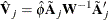whereis the dispersion parameter estimate andis the weight matrix from the final local scoring iteration. If you specify UCLM, LCLM, and STD options in the OUTPUT statement, the statistics are derived based on.

When you request both the ADDITVE and CLM suboptions in the PLOTS=COMPONENTS option, each of the smoothing component plots displays confidence intervals for total prediction of each loess smoother. The confidence intervals are derived from the total variance that is contributed by both the linear and nonlinear parts by the jth term